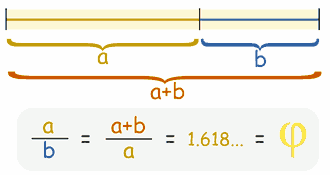# briefly explain the concept pf golden ratio.what do you mean by Klein Bottle.AKASH GOYAL AskiitiansExpert-IITD
419 Points
12 years ago

Dear Tejas

If you divide a line into two parts so that:

 the longer part divided by the smaller part is also equal to the whole length divided by the longer part

then you will have the golden ratio.The Klein bottle is a well-known and interesting surface which, like the Möbius strip, is non-orientable. There are actually two forms of Klein bottles; the one above (parameterized by the equations below) is defined much like a Möbius strip, while the one pictured below, which is defined more topologically, is the variety first proposed by C.F. Klein, for whom the surface is named.

All the best.

AKASH GOYAL